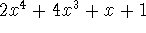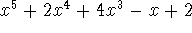CS 2005 Techniques of Programming WPI, B Term 1994
Craig E. Wills Exam 1 (100 pts)
Given: Friday, Nov 11, 1994

NAME: LAB TIME:

1.5em 1.5em

This is a closed book (and notes) examination. Answer all questions on the exam itself. Take the number of points assigned to each problem and the amount of space provided for your answer as a measure of the length and difficulty of the expected solution. The exam totals 100 points.

SCORE:

1. (15 points) Indicate whether the following statements are true or false (write True or False, not T or F).

A stack exhibits FIFO behavior.
To check if two strings are equal in C be sure to use ``=='' rather than ``=''.
An array of characters is the same as a string in C.
The sizeof function in C returns the value of the variable passed to it.
When dynamically allocating space for a string you should always allocate one more byte than the length of the string.

2. (12 points) Consider the following declarations:

`int a, *pa;`

For each scanf() statement below indicate whether it would or would not work correctly to read an integer value if the statement `a = 0;` was initially executed.

```scanf("%d", &a);

scanf("%d", pa);

scanf("%d", *pa);```

For each scanf() statement below indicate whether it would or would not work correctly to read an integer value if the statement `pa = &a;` was initially executed.

```scanf("%d", &a);

scanf("%d", pa);

scanf("%d", *pa);```

3. (10 points) Given the following definitions and variables:

```typedef struct foo {
int w;
char ch;
int rgInt;
struct foo *pFoo;
} FooType;

FooType rgFoo, foo, *pFoo;

pFoo = (FooType *)malloc(sizeof(FooType));```

What is the type of each of the following expressions? Note: some may result in errors.

1. `pFoo`

2. `rgFoo->ch`

3. `foo.rgInt;`

4. `pFoo->rgInt`

5. `foo.w`

4. (8 points) Consider the following Makefile:

```text.o: text.c stack.h text.h
gcc -c text.c -o text.o

stack.o: stack.c stack.h
gcc -c stack.c -o stack.o

text: text.o stack.o
gcc -o text text.o stack.o```

Assume that the files stack.c, text.c, stack.h and text.h were created then the following make command is executed. Next the file text.h is modified. What compilations will occur when the same make command is reexecuted?

`> make text`

5. (12 points) Assume the following procedures have been defined along with type definitions for Stack and Queue:

• InitStack(&s)--initialize a stack of integers

• Push(i, &s)--push an integer onto the stack

• Pop(&i, &s)--pop an integer from the stack into variable i. Return -1 on error, 0 on success.

• InitQueue(&q)--initialize a queue of integers

• DeleteQueue(&i, &q)--delete an integer from the queue and return in variable i. Return -1 on error, 0 on success.

What is the output of the following program segment? For partial credit, show the contents of the stacks and the queue after each loop has executed (be sure and clearly label the stacks and queue).

```    Stack s1, s2;
Queue q;
int i, j;

InitStack(&s1);
InitStack(&s2);
InitQueue(&q);

for (i = 1; i <= 6; i++) {
if (i%2 == 0)
Push(i, &s1);
else
Push(i, &s2);
}

while (Pop(&j, &s1) >= 0) {
if (j%3 == 0)
printf("%d ", j);
else
}

while (Pop(&j, &s2) >= 0)
printf("%d ", j);
while (DeleteQueue(&j, &q) >= 0)
printf("%d ", j);
printf("\n");```

6. (18 points) Consider an array implementation of a queue with the following declarations.

```#define MAXQUEUE 10

typedef struct queue {
int rear;        /* last slot used for insertion */
int front;       /* next slot to delete */
int count;       /* count of items in queue */
Item_type rgItem[MAXQUEUE];
} Queue;```

Using these declarations show the code for AddQueue(), which adds the next element to the queue.

```/*
* AddQueue - add an item to the queue, return zero for success, -1 on error.
*/
{
/* your code goes here */
}```

7. (10 points) Consider an array implementation of a stack with the following declarations:

```#define MAXSTACK 10

typedef struct stack {
int top;        /* top of stack */
Item_type rgItem[MAXSTACK];
} Stack;```

Using these declarations show the code for Peek(), which like Pop() returns the top element of the stack, but unlike Pop() does not actually remove the element from the stack.

```/*
* Peek - peek at top item on the stack, return zero for success, -1 on error.
*/
int Peek(Item_type *pitem, Stack *pstack)
{
/* your code goes here */
}```

8. (15 points) Write a procedure AddPoly() that takes two polynomials and adds them together. Adding polynomials means adding together the like terms. Thus adding togetherandwill yield.

You must use the following declaration for storing a polynomial. Also shown is the declaration of three polynomial variables and calling ReadPoly() to read in two of the polynomials.

```#define MAXCOEFF 10

typedef struct polytype {
int wDegree;
float rgCoeff[MAXCOEFF];
} Polynomial;